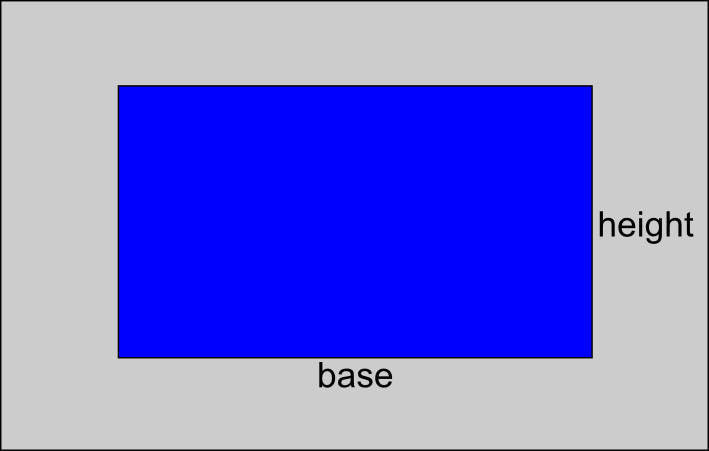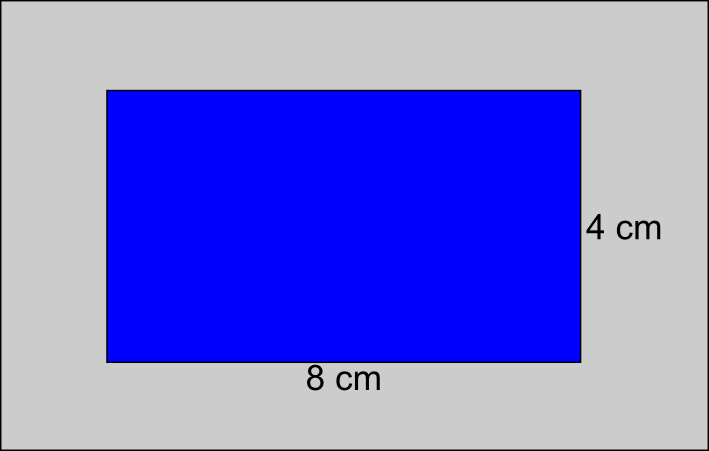The Singing Hedgehog Guide to:
Area - Rectangles

The area of any shape is found by creating a suitable rectangle.
[This is even true for circles!]To work out the area of a rectangle we use a simple formula:

area = base x height

So for this rectangle:area = 8 x 4 = 32 cm²

[don't forget area will always be in SQUARE units]

next page